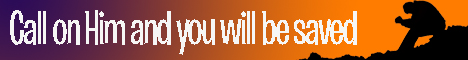Note:  Do not rely on this information. It is very old.

# Trigonometry

Trigonometry derives its name from two Greek words meaning to measure a triangle and originally that was the object of the science. Now, however, its range is much wider; it deals with angles and their measures, with their geometrical properties, and with algebraical investigations in which they are concerned. Angles are measured in three different ways, according to the unit selected. In the English system a right angle is divided into 90 equal parts, each of which is called a degree, and the degree is the unit of angular measurement. The French divide the right angle into 100 parts, and denote the unit the grade. A third system, however, exists, which does not depend upon any arbitrary mode of division. Thus if O be the centre of a circle (Fig. 1), and the arc A B be taken, equal to the radius O, A, the angle A O B is the unit of this system, and is sometimes called a radian._ Any angle may be expressed in terms of this unit, and the result will be the circular measure of the angle. The radian is equivalent to 57.2957 . . . degrees. The number of radians contained in any angle is easily seen to be the length of the arc of any circle subtended by the angle (placed at the centre), divided by the length of the radius of the circle. Thus the circular measure of A 0 C is A C / O A.

Trigonometrical calculations with regard to an angle are generally made with the use of certain ratios between the sides of a right-angled triangle containing the angle, these ratios being known as the trigonometrical functions of the angle. In former times, however, these functions had a somewhat different meaning. Let P A be an arc of a circle whose centre is O (Fig. 2). Produce O P, draw P M perpendicular to O A, and draw A B, the tangent, at A. Let O C be perpendicular to O A, and let the tangent at C meet O P produced at T. Then P M was called the sine of the arc P A, 0 M its cosine, B A its tangent, T C its cotangent, O B its secant, O T its cosecant, and M A (or 1 - cosine) its versed sine. In modern trigonometry these terms are applied to the ratios between the lines of the triangleO P M containing the angle P O M. Thus P M / O P is the sine of the angle P 0 M, O M / O P is its cosine, P M / O M is its tangent, O M / P M its cotangent, O P / O M its secant, and O P / P M its cosecant. It is seen that the lengths of the lines in the old definitions varied with the circle taken, but the modern functions are independent of any radius. If the lengths of the lines representing the old function be divided by the length of the radius of the circle, we see that the old functions of the arc are transformed into the modern functions of the angle. The sine of the angle A is generally written sin A, and the square of this is denoted by sin 2A, similar abbreviations being used for the other functions.

The trigonometrical ratios are connected together by the formulae sin 2A + cos 2A = 1, sec 2A = 1 + tan 2A and cosec 2A = 1 + cot 2A, so that all the ratios of an angle can be expressed in terms of any one of them.

The angle P O C, the amount by which P O M differs from a right angle, is called the complement of P O M and P 0 A1; the difference between it and two right angles is called its supplement. The functions of an angle are always the co-functions of its complement, e.g. sin A = cos (90 - A), while the functions of an angle and its supplement are either equal or differ only in sign.

Geometrically, we can only find the functions of angles of 30°, 45°, 60°, and 72°, but formulae have been arrived at by which the functions of all angle A + B or A - B can be obtained from the functions of the two angles A and B. It follows that the functions of 2A, 3A, etc., and in the same way A/2, A/4, etc., can be found, and so the functions of any angle can be obtained. The solution of triangles is an important branch of trigonometry, on account of its application in mensuration and surveying, and different methods are used, according to the data given, a fundamental proposition connecting the sides and angles of any triangle being that the sides are proportional to the sides of the opposite angles. On the more theoretical side, trigonometry concerns itself with the summation of sines, whose terms are related to the trigonometrical ratios, with the expansion of certain expressions, such as the expansion of sin a and v cos a, in series of powers of a (given in circular measure), with the evaluation of pi (the ratio between circumference and diameter of a circle), and with innumerable other achievements of mathematical importance. Spherical trigonometry is that branch of the science which is applied to the investigation of triangles drawn upon a sphere, and finds its special application in astronomy.